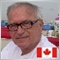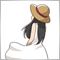# MA20 of ATR10 wrong values returned?27

I'm new to MQL. I'm trying to get the SMA of the ATR value.

Is this supposed to work?

int lookback = 1000;

for(int i=lookback-1;i>=0;i--) atr[i] = iATR(NULL, 0, 10, i);

for(int a=lookback-1;a>=0;a--) ma[a]=iMAOnArray(atr,lookback,20,0,MODE_SMA,a);

Print("atr= "+DoubleToStr(atr, _Digits)+"   ma= "+DoubleToStr(ma, _Digits));

atr is correct... ma is WRONG!

Any assistance would be greatly appreciated. Thanks in advance.

Robert525

What do you mean?

"MA20 of ATR10" is calculated as follows.

MA20 = (ATR + ATR + ATR...... + ATR) / 20;

I checked it by programming, and the results are the same.
(Look at the "Comment". MA1 is the result of "iMAOnArray", and MA2 is the result of the above calculation.)
Files:27

What do you mean?

"MA20 of ATR10" is calculated as follows.

MA20 = (ATR + ATR + ATR...... + ATR) / 20;

I checked it by programming, and the results are the same.
(Look at the "Comment". MA1 is the result of "iMAOnArray", and MA2 is the result of the above calculation.)

Thank you very much Naguisa. You actually answered my "basic" question by explaining that the iMAOnArray can be used ONLY with indicator data! I was trying to use it by simply copying the ATR data to an array atr, then use the "data" as an input to the iMAOnArray an put the SMA results in the ma array. Apparently, that will NOT work.

Thanks again.

Robert